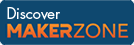Cody

# Problem 44319. Write c^3 as sum of two squares a^2+b^2

Created by Mehmet OZC in Community

write c^3 as sum of two squares a^2+b^2. a and b must be integer and greater than zero.

For example

``` 5^3 = 2^2 + 11^2
5^3 = 5^2 + 10^2
10^3 = 10^2 + 30^2
10^3 = 18^2 + 26^2```

sort output matrix so that each row and first column is in ascending order.

### Solution Stats

34.89% Correct | 65.11% Incorrect
Last solution submitted on Mar 17, 2019

#### TagsMATLAB and Simulink resources for Arduino, LEGO, and Raspberry Pi